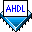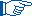## Assigning State Machine Bits & Values

 See AlsoA state bit is an output of a flipflop used by a state machine to store one bit of the value of the state machine. In most cases, you should allow the Compiler to assign state bits and values to minimize the logic resources required: the Logic Synthesizer automatically minimizes the number of state bits needed, optimizing both device utilization and performance.

However, some state machines may operate faster with state values that use more than the minimum number of state bits. In addition, you may want explicit state bits to be the outputs of a state machine. To control these cases, you can declare state machine bits and values in the State Machine Declaration.You can set the State Machine Processing logic option to One-Hot, which implements one-hot encoding for a project. One-hot encoding automatically assigns all state bits to valid states. If you explicitly assign state bits in addition to using one-hot encoding, the project's logic may be implemented inefficiently.

The stepper.tdf file shown below implements a stepper motor controller.

```SUBDESIGN stepper
(
clk, reset   : INPUT;
ccw, cw      : INPUT;
phase[3..0]  : OUTPUT;
)
VARIABLE
ss: MACHINE OF BITS (phase[3..0])
WITH STATES  (
s0 = B"0001",
s1 = B"0010",
s2 = B"0100",
s3 = B"1000");
BEGIN
ss.clk   = clk;
ss.reset = reset;

TABLE
ss,   ccw,   cw   =>   ss;

s0,   1,      x   =>   s3;
s0,   x,      1   =>   s1;
s1,   1,      x   =>   s0;
s1,   x,      1   =>   s2;
s2,   1,      x   =>   s1;
s2,   x,      1   =>   s3;
s3,   1,      x   =>   s2;
s3,   x,      1   =>   s0;
END TABLE;
END;
```

In this example, the `phase[3..0]` outputs declared in the Subdesign Section are also declared as bits of the state machine `ss` in the State Machine Declaration. Note that `ccw` and `cw` must never both be equal to 1 in the same table. AHDL assumes that only one condition in a truth table is true at a time; therefore, overlapping bit patterns may cause unpredictable results. Refer to Truth Table Statement Rules for more information.